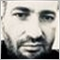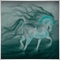# Position size (I don't understand where I go wrong)307

Hello, I am asking for help in understanding where I am making a mistake.

`I want to classically calculate the lot size based on the% risk with equity.`
I read the threads on the forum, tried in my code, but something counts wrong, the "lot" amount is too large (?) but I don't understand something. My code:

```void Buy(MqlRates &pPrice[])
{

double Equity = AccountInfoDouble(ACCOUNT_EQUITY);

double StopLossDistance = atPrice - pPrice.low;

double StopLevelPrice = NormalizeDouble(atPrice - pSLmultiplier * StopLossDistance, _Digits);

double TakeProfit1 = NormalizeDouble(atPrice + StopLossDistance * pTP1multiplier, _Digits);

double valueToRisk = pRisk / 100 * Equity;

double tickCount = StopLossDistance  / tickSize;

double Lots = NormalizeDouble((valueToRisk / (tickCount * tickValue)), 2);

Print("Equity = ", Equity);
Print("Buy at price = ", atPrice);
Print("Stop Loss Distance = ", StopLossDistance);
Print("Stop Loss price = ", StopLevelPrice);
Print("Take Profit price = ", TakeProfit1);
Print("Tick Size = ", tickSize);
Print("Tick Value = ", tickSize);
Print("Risk = ", Risk);
Print("Equity Risk = ", valueToRisk);
Print("Tick Count = ", tickCount);
Print("_Digits = ", _Digits);
Print("_Points = ", _Point);
Print("Lots = ", Lots);

}```

Activity log

I'm definitely doing something wrong, or I'm wrong. I read the threads on the forum and tried to figure it out, but I can't. I have a feeling. Please help.6215

```double Lots = NormalizeDouble((valueToRisk / (tickCount * tickValue)), 2);
```
Is that only calculate method?
If Yes where is the account equity in it.307

Ahmet Metin Yilmaz:
Is that only calculate method?
If Yes where is the account equity in it.

valueToRisk is the variable calculated according to

`double valueToRisk = pRisk / 100 * Equity`307

I think I found a problem. Most likely the "lot" amount is counting correctly (to be confirmed)

But surely there is not enough margin, I add the calculation of the required margin and with the selected % of risk, available margin on the account and the leverage, there is simply no margin.

I reduced the risk level by 10x and now it's ok.

I use 1:25 leverage so I just have to trade with much less risk based on equity.

It is necessary to introduce control of the required margin versus the available margin.22726

1. Risk depends on your initial stop loss, lot size, and the value of the symbol. It does not depend on margin and leverage. No SL means you have infinite risk. Never risk more than a small percentage of your trading funds, certainly less than 2% per trade, 6% total.

1. You place the stop where it needs to be — where the reason for the trade is no longer valid. E.g. trading a support bounce the stop goes below the support.

2. AccountBalance * percent/100 = RISK = OrderLots * (|OrderOpenPrice - OrderStopLoss| * DeltaPerLot + CommissionPerLot) (Note OOP-OSL includes the spread, and DeltaPerLot is usually around \$10/pip but it takes account of the exchange rates of the pair vs. your account currency.)

3. Do NOT use TickValue by itself - DeltaPerLot and verify that MODE_TICKVALUE is returning a value in your deposit currency, as promised by the documentation, or whether it is returning a value in the instrument's base currency.
MODE_TICKVALUE is not reliable on non-fx instruments with many brokers - MQL4 programming forum 2017.10.10
Is there an universal solution for Tick value? - Currency Pairs - General - MQL5 programming forum 2018.02.11
Lot value calculation off by a factor of 100 - MQL5 programming forum 2019.07.19

4. You must normalize lots properly and check against min and max.

5. You must also check FreeMargin to avoid stop out

Most pairs are worth about \$10 per PIP. A \$5 risk with a (very small) 5 PIP SL is \$5/\$10/5 or 0.1 Lots maximum.